## Soddy CirclesGiven three distinct points,, and, let three Circles be drawn, one centered about each point and each one tangent to the other two. Call the Radii(,,). Then the Circles satisfy(1)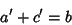(2)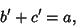(3)

as shown in the diagram below.Solving for the Radii then gives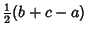(4)(5)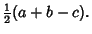(6)

The above Triangle has sides,, and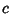, and Semiperimeter(7)

Plugging in,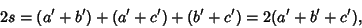(8)

giving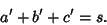(9)(10)

Switchingandto opposite sides of the equation and noting that the above argument applies equally well toand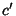then gives(11)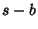(12)(13)

As can be seen from the first figure, there exist exactly two nonintersecting Circles which are Tangent to all three Circles. These are called the inner and outer Soddy circles (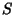and, respectively), and their centers are called the inner and outer Soddy Points.

The inner Soddy circle is the solution to the Four Coins Problem. The centerof the inner Soddy circle is the Equal Detour Point, and the center of the outer Soddy circleis the Isoperimetric Point (Kimberling 1994).

Frederick Soddy (1936) gave the Formula for finding the Radii of the Soddy circles (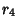) given the Radii(, 2, 3) of the other three. The relationship is(14)

where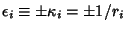are the so-called Bends, defined as the signed Curvatures of the Circles. If the contacts are all external, the signs are all taken as Positive, whereas if one circle surrounds the other three, the sign of this circle is taken as Negative (Coxeter 1969). Using the Quadratic Formula to solve for, expressing in terms of radii instead of curvatures, and simplifying gives(15)

Here, the Negative solution corresponds to the outer Soddy circle and the Positive one to the inner Soddy circle.

This Formula is called the Descartes Circle Theorem since it was known to Descartes.However, Soddy also extended it to Spheres. Gosper has further extended the result tomutually tangent-D Hyperspheres, whose Curvatures satisfy(16)

Solving forgives(17)

For (at least)and 3, the Radical equals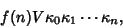(18)

whereis the Content of the Simplex whose vertices are the centers of theindependent Hyperspheres. The Radicand can also become Negative, yielding an Imaginary. For, this corresponds to a sphere touching three large bowling balls and a small BB, all mutually tangent, which is an impossibility.

Bellew has derived a generalization applicable to a Circle surrounded byCircles which are, in turn, circumscribed by another Circle. The relationship is(19)
where(20)

For, this simplifies to the Soddy formula.

See also Apollonius Circles, Apollonius' Problem, Arbelos, Bend (Curvature), Circumcircle, Descartes Circle Theorem, Four Coins Problem, Hart's Theorem, Pappus Chain, Sphere Packing, Steiner Chain

References

Coxeter, H. S. M. Introduction to Geometry, 2nd ed. New York: Wiley, pp. 13-14, 1969.

Elkies, N. D. and Fukuta, J. Problem E3236 and Solution.'' Amer. Math. Monthly 97, 529-531, 1990.

Kimberling, C. Central Points and Central Lines in the Plane of a Triangle.'' Math. Mag. 67, p. 181, 1994.

The Kiss Precise.'' Nature 139, 62, 1937.

Soddy, F. The Kiss Precise.'' Nature 137, 1021, 1936.

Vandeghen, A. Soddy's Circles and the De Longchamps Point of a Triangle.'' Amer. Math. Monthly 71, 176-179, 1964.